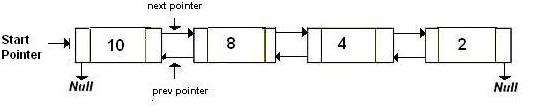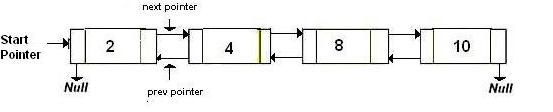# Reverse a Doubly linked list using recursion

Given a doubly linked list. Reverse it using recursion.

`Original Doubly linked list ``Reversed Doubly linked list `## Recommended: Please try your approach on {IDE} first, before moving on to the solution.

We have discussed
Iterative solution to reverse a Doubly Linked List

Algorithm
1) If list is empty, return
3) If prev = NULL it means that list is fully reversed. Else reverse(head->prev)

## C++

 `// C++ implementation to reverse a doubly ` `// linked list using recursion ` `#include ` `using` `namespace` `std; ` ` `  `// a node of the doubly linked list ` `struct` `Node { ` `    ``int` `data; ` `    ``Node *next, *prev; ` `}; ` ` `  `// function to get a new node ` `Node* getNode(``int` `data) ` `{ ` `    ``// allocate space ` `    ``Node* new_node = ``new` `Node; ` `    ``new_node->data = data; ` `    ``new_node->next = new_node->prev = NULL; ` `    ``return` `new_node; ` `} ` ` `  `// function to insert a node at the beginning ` `// of the Doubly Linked List ` `void` `push(Node** head_ref, Node* new_node) ` `{ ` `    ``// since we are adding at the beginning, ` `    ``// prev is always NULL ` `    ``new_node->prev = NULL; ` ` `  `    ``// link the old list off the new node ` `    ``new_node->next = (*head_ref); ` ` `  `    ``// change prev of head node to new node ` `    ``if` `((*head_ref) != NULL) ` `        ``(*head_ref)->prev = new_node; ` ` `  `    ``// move the head to point to the new node ` `    ``(*head_ref) = new_node; ` `} ` ` `  `// function to reverse a doubly linked list ` `Node* Reverse(Node* node) ` `{ ` `    ``// If empty list, return ` `    ``if` `(!node) ` `        ``return` `NULL; ` ` `  `    ``// Otherwise, swap the next and prev ` `    ``Node* temp = node->next; ` `    ``node->next = node->prev; ` `    ``node->prev = temp; ` ` `  `    ``// If the prev is now NULL, the list ` `    ``// has been fully reversed ` `    ``if` `(!node->prev) ` `        ``return` `node; ` ` `  `    ``// Otherwise, keep going ` `    ``return` `Reverse(node->prev); ` `} ` ` `  `// Function to print nodes in a given doubly ` `// linked list ` `void` `printList(Node* head) ` `{ ` `    ``while` `(head != NULL) { ` `        ``cout << head->data << ``" "``; ` `        ``head = head->next; ` `    ``} ` `} ` ` `  `// Driver program to test above ` `int` `main() ` `{ ` `    ``// Start with the empty list ` `    ``Node* head = NULL; ` ` `  `    ``// Create doubly linked: 10<->8<->4<->2 */ ` `    ``push(&head, getNode(2)); ` `    ``push(&head, getNode(4)); ` `    ``push(&head, getNode(8)); ` `    ``push(&head, getNode(10)); ` `    ``cout << ``"Original list: "``; ` `    ``printList(head); ` ` `  `    ``// Reverse doubly linked list ` `    ``head = Reverse(head); ` `    ``cout << ``"\nReversed list: "``; ` `    ``printList(head); ` `    ``return` `0; ` `} `

## Java

 `// Java implementation to reverse a doubly  ` `// linked list using recursion  ` `class` `GFG ` `{ ` `     `  `// a node of the doubly linked list  ` `static` `class` `Node  ` `{  ` `    ``int` `data;  ` `    ``Node next, prev;  ` `};  ` ` `  `// function to get a new node  ` `static` `Node getNode(``int` `data)  ` `{  ` `    ``// allocate space  ` `    ``Node new_node = ``new` `Node();  ` `    ``new_node.data = data;  ` `    ``new_node.next = new_node.prev = ``null``;  ` `    ``return` `new_node;  ` `}  ` ` `  `// function to insert a node at the beginning  ` `// of the Doubly Linked List  ` `static` `Node push(Node head_ref, Node new_node)  ` `{  ` `    ``// since we are adding at the beginning,  ` `    ``// prev is always null  ` `    ``new_node.prev = ``null``;  ` ` `  `    ``// link the old list off the new node  ` `    ``new_node.next = (head_ref);  ` ` `  `    ``// change prev of head node to new node  ` `    ``if` `((head_ref) != ``null``)  ` `        ``(head_ref).prev = new_node;  ` ` `  `    ``// move the head to point to the new node  ` `    ``(head_ref) = new_node;  ` `    ``return` `head_ref; ` `}  ` ` `  `// function to reverse a doubly linked list  ` `static` `Node Reverse(Node node)  ` `{  ` `    ``// If empty list, return  ` `    ``if` `(node == ``null``)  ` `        ``return` `null``;  ` ` `  `    ``// Otherwise, swap the next and prev  ` `    ``Node temp = node.next;  ` `    ``node.next = node.prev;  ` `    ``node.prev = temp;  ` ` `  `    ``// If the prev is now null, the list  ` `    ``// has been fully reversed  ` `    ``if` `(node.prev == ``null``)  ` `        ``return` `node;  ` ` `  `    ``// Otherwise, keep going  ` `    ``return` `Reverse(node.prev);  ` `}  ` ` `  `// Function to print nodes in a given doubly  ` `// linked list  ` `static` `void` `printList(Node head)  ` `{  ` `    ``while` `(head != ``null``) ` `    ``{  ` `        ``System.out.print( head.data + ``" "``);  ` `        ``head = head.next;  ` `    ``}  ` `}  ` ` `  `// Driver code  ` `public` `static` `void` `main(String args[])  ` `{  ` `    ``// Start with the empty list  ` `    ``Node head = ``null``;  ` ` `  `    ``// Create doubly linked: 10<.8<.4<.2 /  ` `    ``head = push(head, getNode(``2``));  ` `    ``head = push(head, getNode(``4``));  ` `    ``head = push(head, getNode(``8``));  ` `    ``head = push(head, getNode(``10``));  ` `    ``System.out.print( ``"Original list: "``);  ` `    ``printList(head);  ` ` `  `    ``// Reverse doubly linked list  ` `    ``head = Reverse(head);  ` `    ``System.out.print(``"\nReversed list: "``);  ` `    ``printList(head);  ` `}  ` `} ` ` `  `// This code is contributed by Arnab Kundu `

## Python3

 `# Python3 implementation to reverse a doubly ` `# linked list using recursion ` `import` `math ` ` `  `# a node of the doubly linked list ` `class` `Node:  ` `    ``def` `__init__(``self``, data):  ` `        ``self``.data ``=` `data  ` `        ``self``.``next` `=` `None` ` `  `# function to get a new node ` `def` `getNode(data): ` `     `  `    ``# allocate space ` `    ``new_node ``=` `Node(data) ` `    ``new_node.data ``=` `data ` `    ``new_node.``next` `=` `new_node.prev ``=` `None` `    ``return` `new_node ` ` `  `# function to insert a node at the beginning ` `# of the Doubly Linked List ` `def` `push(head_ref, new_node): ` `     `  `    ``# since we are adding at the beginning, ` `    ``# prev is always None ` `    ``new_node.prev ``=` `None` ` `  `    ``# link the old list off the new node ` `    ``new_node.``next` `=` `head_ref ` ` `  `    ``# change prev of head node to new node ` `    ``if` `(head_ref !``=` `None``): ` `        ``head_ref.prev ``=` `new_node ` ` `  `    ``# move the head to point to the new node ` `    ``head_ref ``=` `new_node ` `    ``return` `head_ref ` ` `  `# function to reverse a doubly linked list ` `def` `Reverse(node): ` `     `  `    ``# If empty list, return ` `    ``if` `not` `node: ` `        ``return` `None` ` `  `    ``# Otherwise, swap the next and prev ` `    ``temp ``=` `node.``next` `    ``node.``next` `=` `node.prev ` `    ``node.prev ``=` `temp ` ` `  `    ``# If the prev is now None, the list ` `    ``# has been fully reversed ` `    ``if` `not` `node.prev: ` `        ``return` `node ` ` `  `    ``# Otherwise, keep going ` `    ``return` `Reverse(node.prev) ` ` `  `# Function to print nodes in a given doubly ` `# linked list ` `def` `printList(head): ` `    ``while` `(head !``=` `None``) : ` `        ``print``(head.data, end ``=` `" "``) ` `        ``head ``=` `head.``next` `     `  `# Driver Code ` `if` `__name__``=``=``'__main__'``:  ` `     `  `    ``# Start with the empty list ` `    ``head ``=` `None` ` `  `    ``# Create doubly linked: 10<.8<.4<.2 */ ` `    ``head ``=` `push(head, getNode(``2``));  ` `    ``head ``=` `push(head, getNode(``4``));  ` `    ``head ``=` `push(head, getNode(``8``));  ` `    ``head ``=` `push(head, getNode(``10``)); ` `    ``print``(``"Original list: "``, end ``=` `"") ` `    ``printList(head) ` `     `  `    ``# Reverse doubly linked list ` `    ``head ``=` `Reverse(head) ` `    ``print``(``"\nReversed list: "``, end ``=` `"") ` `    ``printList(head) ` `     `  `# This code is contributed by Srathore `

## C#

 `// C# implementation to reverse a doubly  ` `using` `System; ` ` `  `// linked list using recursion  ` `class` `GFG  ` `{  ` `     `  `// a node of the doubly linked list  ` `public` `class` `Node  ` `{  ` `    ``public` `int` `data;  ` `    ``public` `Node next, prev;  ` `};  ` ` `  `// function to get a new node  ` `static` `Node getNode(``int` `data)  ` `{  ` `    ``// allocate space  ` `    ``Node new_node = ``new` `Node();  ` `    ``new_node.data = data;  ` `    ``new_node.next = new_node.prev = ``null``;  ` `    ``return` `new_node;  ` `}  ` ` `  `// function to insert a node at the beginning  ` `// of the Doubly Linked List  ` `static` `Node push(Node head_ref, Node new_node)  ` `{  ` `    ``// since we are adding at the beginning,  ` `    ``// prev is always null  ` `    ``new_node.prev = ``null``;  ` ` `  `    ``// link the old list off the new node  ` `    ``new_node.next = (head_ref);  ` ` `  `    ``// change prev of head node to new node  ` `    ``if` `((head_ref) != ``null``)  ` `        ``(head_ref).prev = new_node;  ` ` `  `    ``// move the head to point to the new node  ` `    ``(head_ref) = new_node;  ` `    ``return` `head_ref;  ` `}  ` ` `  `// function to reverse a doubly linked list  ` `static` `Node Reverse(Node node)  ` `{  ` `    ``// If empty list, return  ` `    ``if` `(node == ``null``)  ` `        ``return` `null``;  ` ` `  `    ``// Otherwise, swap the next and prev  ` `    ``Node temp = node.next;  ` `    ``node.next = node.prev;  ` `    ``node.prev = temp;  ` ` `  `    ``// If the prev is now null, the list  ` `    ``// has been fully reversed  ` `    ``if` `(node.prev == ``null``)  ` `        ``return` `node;  ` ` `  `    ``// Otherwise, keep going  ` `    ``return` `Reverse(node.prev);  ` `}  ` ` `  `// Function to print nodes in a given doubly  ` `// linked list  ` `static` `void` `printList(Node head)  ` `{  ` `    ``while` `(head != ``null``)  ` `    ``{  ` `        ``Console.Write( head.data + ``" "``);  ` `        ``head = head.next;  ` `    ``}  ` `}  ` ` `  `// Driver code  ` `public` `static` `void` `Main(String []argsS)  ` `{  ` `    ``// Start with the empty list  ` `    ``Node head = ``null``;  ` ` `  `    ``// Create doubly linked: 10<.8<.4<.2 /  ` `    ``head = push(head, getNode(2));  ` `    ``head = push(head, getNode(4));  ` `    ``head = push(head, getNode(8));  ` `    ``head = push(head, getNode(10));  ` `    ``Console.Write( ``"Original list: "``);  ` `    ``printList(head);  ` ` `  `    ``// Reverse doubly linked list  ` `    ``head = Reverse(head);  ` `    ``Console.Write(``"\nReversed list: "``);  ` `    ``printList(head);  ` `}  ` `}  ` ` `  `// This code is contributed by Arnab Kundu  `

Output:

```Original list: 10 8 4 2
Reversed list: 2 4 8 10
```

My Personal Notes arrow_drop_upCheck out this Author's contributed articles.

If you like GeeksforGeeks and would like to contribute, you can also write an article using contribute.geeksforgeeks.org or mail your article to contribute@geeksforgeeks.org. See your article appearing on the GeeksforGeeks main page and help other Geeks.

Please Improve this article if you find anything incorrect by clicking on the "Improve Article" button below.

Improved By : andrew1234, sapnasingh4991